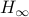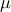# Fast Evaluation of Quadratic Control-Lyapunov Policy

Y. Wang and S. Boyd

IEEE Transactions on Control Systems Technology, 19(4):939–946, July 2011.

The evaluation of a control-Lyapunov policy, with quadratic Lyapunov function, requires the solution of a quadratic program (QP) at each time step. For small problems this QP can be solved explicitly; for larger problems an on-line optimization method can be used. For this reason the control-Lyapunov control policy is considered a computationally intensive control law, as opposed to an ‘analytical’ control law, such as conventional linear state feedback, LQG, or, too complex or slow to be used in high speed control applications. In this note we show that by pre-computing certain quantities, the control-Lyapunov policy can be evaluated extremely efficiently. We will show that when the number of inputs is on the order of the square-root of the state dimension, the cost of evaluating a control-Lyapunov policy is on the same order as the cost of evaluating a simple linear state feedback policy, and less (in order) than the cost of updating a Kalman filter state estimate. To give an idea of the speeds involved, for a problem with 100 states and 10 inputs, the control-Lyapunov policy can be evaluated in around 67s, on a 2GHz AMD processor; the same processor requires 44s to carry out a Kalman filter update.